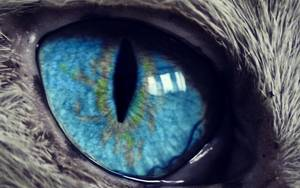# Transformations

There are two kinds of transformations: ones that actually modify the image and others that change the context of further transformations down the manipulation chain. You'll find both types listed here, in alphabetical order, each with a short description of its specific syntax and behavior.

## contain

Syntax: `contain=<size>`

contain behaves like the CSS background size "contain". It will resize the image so that it completely fits inside the target area while conserving the original aspect ratio. The resulting image will be smaller than a target size which aspect ratio is not the same as the aspect ratio of the input.

For instance, applying `contain=150x100` to our beloved face of a cat will result in the following 150x94 image:

Result## contain-max

Syntax: `contain-max=<pixel size>`

A conditional version of contain that will be applied only when one of the given lengths is smaller than the corresponding input image dimension.

## contain-min

Syntax: `contain-min=<pixel size>`

A conditional version of contain that will be applied only when one of the given lengths is larger than the corresponding input image dimension.

## cover

Syntax: `cover=<size|ratio>`

cover behaves like the CSS background size "cover". It will resize the image so that it completely fills the target area while conserving the original aspect ratio. If some parts of the image end up outside of the covered area, they are cropped.

So, a `cover=100x100` of a 300x188 image would first scale the image down to a height of 100 pixels and then crop along the x-axis as demonstrated below:

Process Result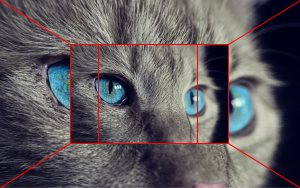cover will use the focus point as a guide and will crop the image so that the focus point is as central as possible in the resulting image. For instance, `focus=85x85/cover=100x100` will behave as follows:

Process ResultWhen a ratio is provided, cover will extract the biggest possible area that satisfies the ratio and is as centered on the focus point as possible. For instance, `focus=85x85/cover=1:1` will behave as follows:

Process Result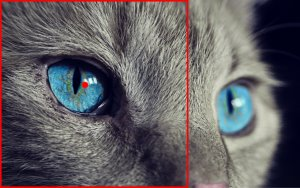## cover-max

Syntax: `cover-max=<pixel size>`

A conditional version of cover that will be applied only when one of the given lengths is smaller than the corresponding input image dimension.

## cover-min

Syntax: `cover-min=<pixel size>`

A conditional version of cover that will be applied only when one of the given lengths is larger than the corresponding input image dimension.

## crop

Syntax: `crop=<crop size>[@<coordinates>]`

crop will extract a zone from the image which size is the given crop size. If no coordinates are given, the focus point will be used as a guide to determine where to start the extraction. If coordinates are given, they will be used to determine the top-left pixel from which to start the extraction.

Here are two examples:

Manipulation Process Result
`crop=200x100`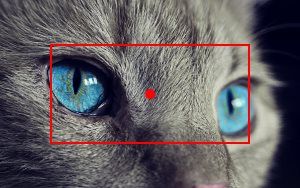`crop=200x100@20x50`## focus

Syntax: `focus=<coordinates>`

focus will set the focus point coordinates. It doesn't modify the output image in any way but will change the behavior of further transformations that take the focus point into account (namely cover, crop and resize).

## format

Syntax: `format=<format expression>`

Specifies the output format. Only the last format in the manipulation expression is taken into account. By default, TwicPics will "smart-guess" the best format for the browser currently requesting the image but you can use format to override this behavior.

Available formats are:

• `jpeg[-<quality>]` for jpeg where quality is an integer between 1 and 100 (default 70)
• `png` for png
• `webp[-<quality>]` for webp where quality is an integer between 1 and 100 (default 70)

## max

Syntax: `max=<pixel size>`

Alias of contain-max.

## min

Syntax: `min=<pixel size>`

Alias of contain-min.

## quality

Syntax: `quality=<quality>`

Specifies the output quality as a number between 1 and 100. Only applies to jpeg and webp outputs.

## quality-max

Syntax: `quality-max=<quality>`

A conditional version of quality that will be applied only when given quality is below current quality.

Manipulation Resulting quality
`quality=100/quality-max=50` `50`
`quality=20/quality-max=50` `20`
`quality-max=50/quality=100` `100`

## quality-min

Syntax: `quality-min=<quality>`

A conditional version of quality that will be applied only when given quality is above current quality.

Manipulation Resulting quality
`quality=100/quality-min=50` `100`
`quality=20/quality-min=50` `50`
`quality-min=50/quality=100` `100`

## resize

Syntax: `resize=<size|ratio>`

resize will resize the image to the specified size. If only one length is provided, the other dimension will be determined so as to respect the aspect ratio of the input image. If both lengths are provided, the aspect ratio may not be respected.

Here are three resize operations on the same source image:

Manipulation Result
`resize=100``resize=-x100`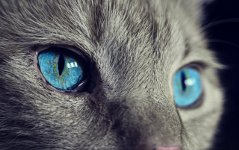`resize=100x100`If a ratio is provided, the image will be resized so that it stays as close as possible to its current surface (i.e number of pixels) while respecting the given aspect ratio as closely as possible.

For instance, `focus=85x85/resize=4:3` will result in the following 274x206 image:

Result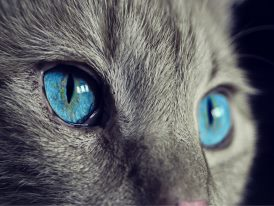The 300x188 image was resized to a 274x206 image (respecting the 4:3 ratio as best as possible). The initial surface was (300x188=) 56,400 pixels. The final surface is (274x206=) 56,444 pixels.

## resize-max

Syntax: `resize-max=<pixel size>`

A conditional version of resize that will be applied only when one of the given lengths is smaller than the corresponding input image dimension.

## resize-min

Syntax: `resize-min=<pixel size>`

A conditional version of resize that will be applied only when one of the given lengths is larger than the corresponding input image dimension.

## step

Syntax: `step=<pixel size>`

step will round the image width and/or height to the closest multiples of the corresponding pixel lengths. If only one length is provided, the other dimension will be determined so as to respect the aspect ratio of the input image. If both lengths are provided, the aspect ratio may not be respected.

Let's take the example of a 206 pixel-wide per 103 pixel-high image (aspect ratio 2:1):

• `step=10` will resize the width to 210 and result in a 210 per 105 output, respecting the 2:1 aspect ratio
• `step=-x10` will resize the height to 100 and result in a 200 per 100 output, respecting the 2:1 aspect ratio
• `step=10x10` will resize both the width to 210 and the height to 100 thus not respecting the 2:1 aspect ratio

## zoom

Syntax: `zoom=<factor>`

Zooms into the image by a factor equal or superior to 1 towards the focus point while preserving the image size.

Factor Result
1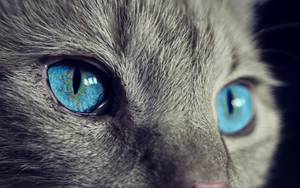1.52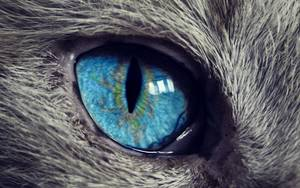3